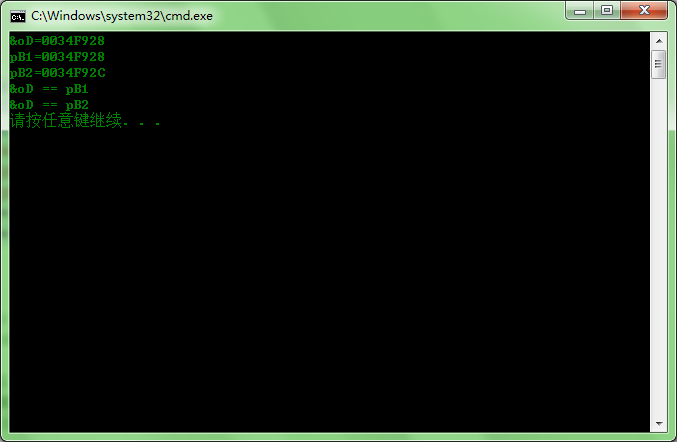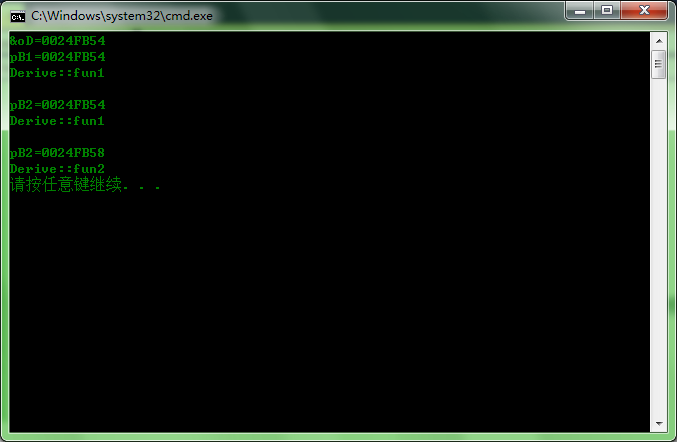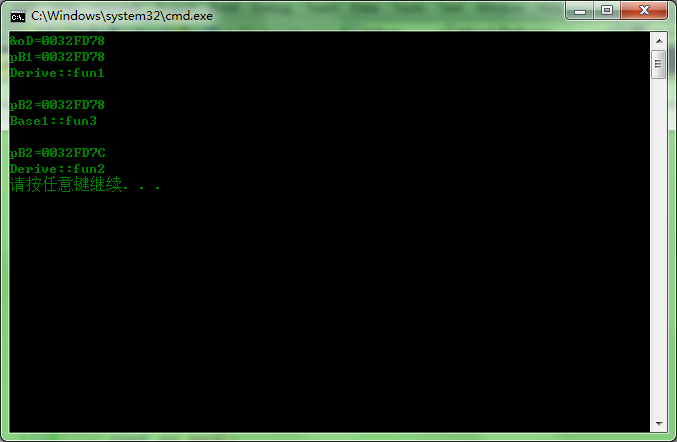# C++多重继承的指针问题

class Base1
{
public:
virtual void fun1() {cout << "Base1::fun1" << endl;};
};

class Base2
{
public:
virtual void fun2() {cout << "Base2::fun1" << endl;};
};

class Derive : public Base1, public Base2
{
public:
virtual void fun1 () {cout << "Derive::fun1" << endl;}
virtual void fun2 () {cout << "Derive::fun2" << endl;}
};

int main()
{
Derive oD;
Base1 *pB1 = (Base1*)(&oD);
Base2 *pB2 = (Base2*)(&oD);
cout << "&oD=" << &oD << '\n';
cout << "pB1=" << pB1 << '\n';
cout << "pB2=" << pB2 << '\n';
if (&oD == pB1) cout << "&oD == pB1" << '\n';
if (&oD == pB2) cout << "&oD == pB2" << '\n';
}Base1 Base2 Derive

int main(){
Derive oD;
cout << "&oD=" << &oD << '\n';

Base1 *pB1 =  &oD;
cout << "pB1=" << pB1 << '\n';
pB1->fun1();
cout << endl;

Base2 *pB2 = (Base2*)(pB1); // 指针强行转换，没有偏移
cout << "pB2=" << pB2 << '\n';
pB2->fun2();
cout << endl;

pB2 = dynamic_cast<Base2*>(pB1); // 指针动态转换,dynamic_cast帮你偏移
cout << "pB2=" << pB2 << '\n';
pB2->fun2();

return 0;
}class Base1
{
public:
virtual void fun3() {cout << "Base1::fun3" << endl;};
virtual void fun1() {cout << "Base1::fun1" << endl;};
};int main()
{
Derive *pD = new Derive();
Base2 *pB2 = pD;
pB2->fun2();
Base1 *pB1 = (Base1*)(pB2);
pB1->fun1();
return 0;
}

1. C++多重继承需要慎用
2. 类型转换尽量采用c++内置的类型转换函数，而不要强行转换。

04-2310-18693
05-09115
04-301607
12-09369
10-24127
04-16608
03-08
09-271092
09-10493
06-06
01-094万+